Sediment Transport
The Sediment Transport category is displayed on the Methods tab, not the Accounting Methods tab.
This method category is available only if the Abiquiu, Cochiti, or Jemez Gain Loss method has been selected for the Reservoir Account Gain Loss category. See Abiquiu, Cochiti, and Jemez Gain Loss.
The sedimentation methods in this category are called only in Accounting; they are not called during physical simulation.Total Vol Sed (Post2000)
This method calculates the sediment calculates the storage volume lost to sedimentation. It calculates sidement load in tons, as a function of the reservoir inflow. It then converts this to a volume based on the sediment density.
Note:  This method is hard-coded to calculate daily sediment load based on units of cfs for the reservoir inflow and tons for the sediment load. The Exponent value in the Seasonal Inflow Coeffs slot must be set accordingly.
This method is currently configured to function correctly for 1 Day and 1 Month timestep sizes only.
At a 1 Month timestep, sediment load is calculated by first finding daily sediment load using the average daily inflow for the month, and then multiplying the daily load by the number of days in the month. The results from a 1 Month timestep may not exactly match the aggregate of the daily results for the month if the daily inflows vary significantly within the month.
Slots Specific to This Method
Type: Table Slot
Units: Flow, None, None
Description: Slope and intercept values for calculating Bed Load at discrete inflow values
Information: The first column contains reservoir inflow values. The second column is the slope and the third column is the intercept used when calculating bed load for a given inflow. The slope and intercept should be based on daily sediment load in tons for an inflow in cfs, regardless of the timestep length or the units set on any other slot or column.The method uses a table lookup to select the slope and intercept value (not interpolation). It uses the coefficient and exponent from the row corresponding to the largest flow value than or equal to the reservoir Inflow
I/O: Required Input
Est Sed Deposition
Type: Table Series Slot
Units: Length, Mass, Mass, None, Volume, Volume
Description: The final outputs from the sediment calculations
Information: The final column, Accumulated Perm Sediment, is the primary output value of this method. The value is also shown on the series slot Accumulated Permanent Sediment, which is used later by accounting methods. Details of the values in this table are given in the method description below.
I/O: Output
Permanent Sediment Content
Type: Table Slot
Units: Volume
Description: The storage volume occupied by permanent sediment
I/O: Required Input
Accumulated Permanent Sediment
Type: Series Slot
Units: Volume
Description: The accumulated storage volume occupied by permanent sediment. Values in this series slot are the same as the values in the Est Sed Deposition slot, Accumulated Perm Sediment column.
Information: If the initial timestep value on this slot is not known, but the value on the table series slot is known, the table series slot value is copied to the series slot at the initial timestep and set as an input.
I/O: Initial Input, remaining values output.
Seasonal Inflow Coeffs
Type: Table Slot
Units: None, Flow, None, None
Description: The coefficient and exponent to use in calculating sediment load for a given month and a given reservoir inflow
The first column contains the month, represented by an integer. At least one row is required for each month. The second column contains inflow values, which should be monotonically increasing for each month. The third column contains coefficient values for each month and specified flow. The fourth column contains exponent values for each month and specified flow. The coefficient and exponent should be based on daily sediment load in tons for an inflow in cfs, regardless of the timestep length or the units set on any other slot or column. The method uses a table lookup to select the coefficient and exponent value (not interpolation). It uses the coefficient and exponent from the row corresponding to the largest flow value for the month less than or equal to the reservoir Inflow.
I/O: Required Input
Sed Data
Type: Table Slot
Units: None, None, Length, None, None, Sed Density
Description: Parameter values used for converting sediment load in tons to an equivalent volume
I/O: Required Input
Method Details
The Total Vol Sed (Post2000) method carries out the following steps.
Calculate the daily inflow volume in cfs‑day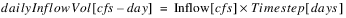IF isMonthly
For a 1 Month timestep the timestep inflow volume must be divided by the number of days in the month to get the daily inflow volume.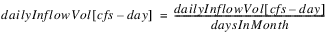END IF
Get the Bed Load Coefficients (bedLoadSlope and bedLoadIntcpt) from the Bed Load Coeffs table slot, corresponding to reservoir Inflow. Use the row for the largest Inflow value less than or equal to the reservoir Inflow.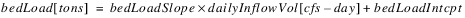Get the Seasonal Inflow Coefficients
Get the Seasonal Inflow Coefficients (sedLoadCoeff, and sedLoadExpon). Use the row for the largest Inflow value less than or equal to the reservoir Inflow.
Calculate the total sediment
1. Calculate total sediment load in tons.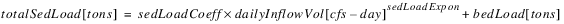IF isMonthly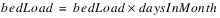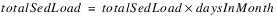END IF
Calculate the elevation of the permanent sediment pool
This calculation will be different for Cochiti than for Abiquiu and Jemez. For Cochiti the calculation depends on whether the Time Varying Elevation Volume method has been selected for the Sediment category. The previous Accumulated Perm Sediment will be added to the sediment content when calculating the permPoolElev for Cochiti unless the Time Varying Elevation Volume method is selected and the current timestep matches the date in a column header of the Elevation Volume Table Time Varying slot (isElevVolModDate = TRUE).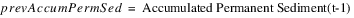IF Reservoir = Abiquiu OR Reservoir = Jemez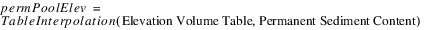ELSE IF Reservoir = Cochiti
IF (Time Varying Elevation Volume is selected) AND (isElevVolModDate = TRUE)ELSE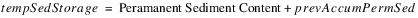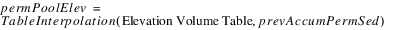END IF
END IF
Calculate the sediment above the perm pool
Calculate the sediment above the perm pool (a percent) and the volume lost to sedimentation at this timestep.
IF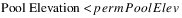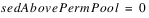ELSE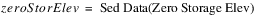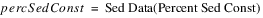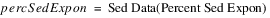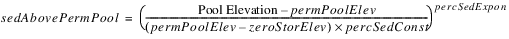END IF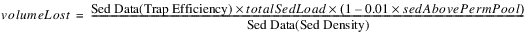Calculate the accumulated permanent sediment
Calculate the accumulated permanent sediment as the previous accumulated sediment plus the volume lost to sedimentation at the current timestep, unless the Time Varying Elevation Volume method is selected and it is an ElevVol Mod Date.
IF (Time Varying Elevation Volume is selected) AND (isElevVolModDate = TRUE)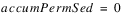ELSE (the typical case)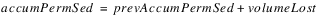END IF
Set all final values in the EST SED Deposition table series slot

Table A.24  Est Sed Deposition
Value
Perm Pool Elev (Length)
permPoolElev